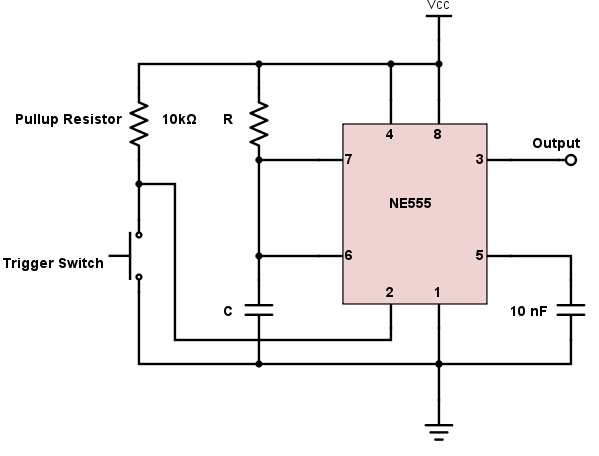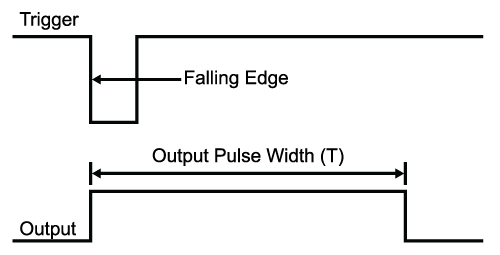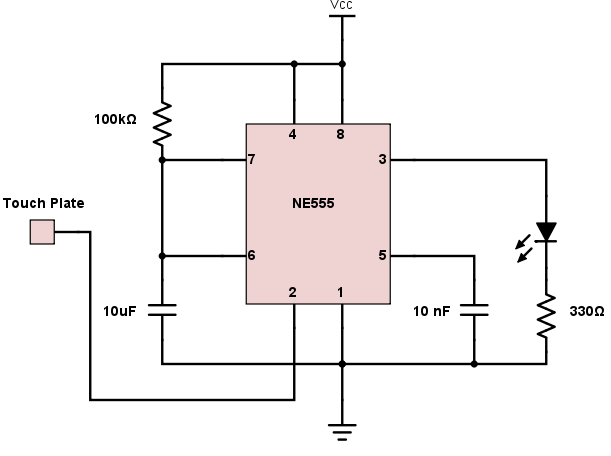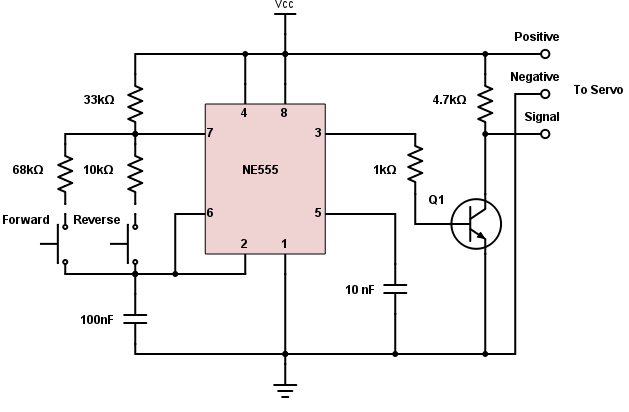# 555 Timer Monostable Circuit Calculator

## This calculator computes for the output pulse width of a 555 timer monostable circuit

### Output

Seconds (s)### Calculating the Output Pulse Width of a Monostable Circuit

The 555 timer above is configured as a monostable circuit. This means that the output voltage becomes high for a set duration (T) when a falling edge is detected on pin 2 (trigger). The circuit above is also called a one-shot circuit. This calculator is designed to compute for the output pulse width of a 555 timer monostable circuit.### The Output Pulse Width Equation

The formula for the Output Pulse Width (T) is given as:

$$T = 1.1 * R * C$$

As shown in the formula, the output pulse width is determined only by the resistor and capacitor combination. This gives the circuit a number of possible applications.

This 555 monostable circuit can generate pulses from a few microseconds to several hours depending on the values of resistor R and capacitor C. Note, however, that the use of very large capacitor (usually electrolytic-type) values are discouraged. This is because of their wide tolerance limits, which means their actual value is far from their marked value. Another issue with such a capacitor is its high leakage current, which may affect the timing accuracy. If a large capacitance is needed, choose a type with a lower leakage current such as tantalum.

Problems may also occur when using small value capacitors for producing very short delays. Stray circuit capacitance can change the value of timing capacitance considerably for values less than 100 pF, which of course leads to inaccurate timing.

### Applications for Monostable Circuits

#### Touch Switch

The monostable circuit above can be used as a simple touch switch. A touch plate can be connected to the trigger pin, which will ground the pin when touched. This will produce a pulse at the output whose width is determined by the R and C combination. A simple touch switch circuit is shown below:Using our calculator, the circuit above will produce a pulse with a width of 1.1 s. This means the LED connected at the output (pin 3) will light for 1.1 s when the plate is touched momentarily.

#### Servo Motor Tester

A servo motor works by accepting pulses with widths from 1 ms to 2 ms. Monostable circuits can be used to test a servo motor by carefully selecting the R and C values to produce the said pulse widths. An example is shown below:• F
f_tassan November 05, 2017

Can someone explain what Vcc does?

Like.
•RK37 November 06, 2017
Sorry, I don't understand your question. Could you be more specific?
Like.
• T
throbscottle January 02, 2018
It is the supply voltage to the circuit. It stands for "Voltage common collector", but just means the positive terminal of your battery or whatever.
Like.